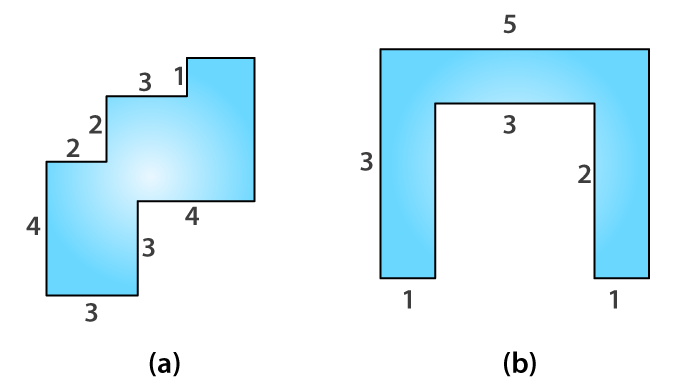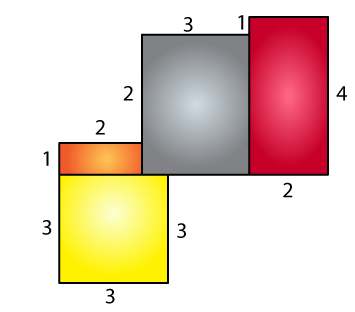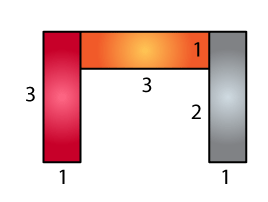# By splitting the following figures into rectangles, find their areas (The measures are given in centimetres).Solution:

(a)Area of yellow region = 3 × 3

= 9 cm2

Area of orange region = 1× 2

= 2 cm2

Area of grey region = 3 × 3

= 9 cm2

Area of brown region = 2 × 4

= 8 cm2

Total area = 9 + 2 + 9 + 8

= 28 cm2

∴ Total area is 28 cm2

(b)Area of brown region = 3 × 1

= 3 cm2

Area of orange region = 3 × 1

= 3 cm2

Area of grey region = 3 × 1

= 3 cm2

Total area = 3 + 3 + 3

= 9 cm2

∴ Total area is 9 cm2(0)(0)